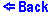A Four-Resistor Circuit

Increase/decrease the magnitude of the EMF and the resistors by clicking on the upper/lower part of the object. The values of the resistors range from 1 to 10 ohms. And the value of the EMF ranges from -10 to +10 Volts. Erase or replace the resistors by selecting the "Eraser" or the wire from the menu. You can place up to four resistors. Resistors can only be placed vertically.
The current flowing through the circuit is defned as:
I = E / R .
The total resistance is determined as the sum of the resistors in series,
Rseries = R1 + R2 ,
and in parallel
Rparallel = R1 * R2 / (R1 + R2) .